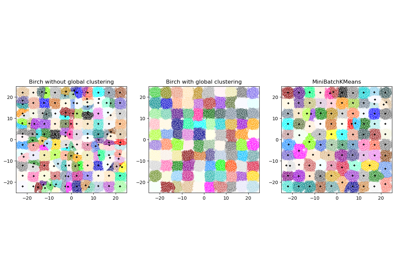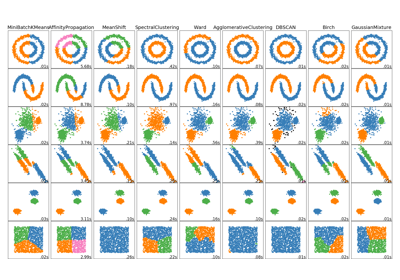# sklearn.cluster.Birch¶

class sklearn.cluster.Birch(threshold=0.5, branching_factor=50, n_clusters=3, compute_labels=True, copy=True)[source]

Implements the Birch clustering algorithm.

It is a memory-efficient, online-learning algorithm provided as an alternative to MiniBatchKMeans. It constructs a tree data structure with the cluster centroids being read off the leaf. These can be either the final cluster centroids or can be provided as input to another clustering algorithm such as AgglomerativeClustering.

Read more in the User Guide.

Parameters: threshold : float, default 0.5 The radius of the subcluster obtained by merging a new sample and the closest subcluster should be lesser than the threshold. Otherwise a new subcluster is started. Setting this value to be very low promotes splitting and vice-versa. branching_factor : int, default 50 Maximum number of CF subclusters in each node. If a new samples enters such that the number of subclusters exceed the branching_factor then that node is split into two nodes with the subclusters redistributed in each. The parent subcluster of that node is removed and two new subclusters are added as parents of the 2 split nodes. n_clusters : int, instance of sklearn.cluster model, default 3 Number of clusters after the final clustering step, which treats the subclusters from the leaves as new samples. None : the final clustering step is not performed and the subclusters are returned as they are. sklearn.cluster Estimator : If a model is provided, the model is fit treating the subclusters as new samples and the initial data is mapped to the label of the closest subcluster. int : the model fit is AgglomerativeClustering with n_clusters set to be equal to the int. compute_labels : bool, default True Whether or not to compute labels for each fit. copy : bool, default True Whether or not to make a copy of the given data. If set to False, the initial data will be overwritten. root_ : _CFNode Root of the CFTree. dummy_leaf_ : _CFNode Start pointer to all the leaves. subcluster_centers_ : ndarray, Centroids of all subclusters read directly from the leaves. subcluster_labels_ : ndarray, Labels assigned to the centroids of the subclusters after they are clustered globally. labels_ : ndarray, shape (n_samples,) Array of labels assigned to the input data. if partial_fit is used instead of fit, they are assigned to the last batch of data.

Notes

The tree data structure consists of nodes with each node consisting of a number of subclusters. The maximum number of subclusters in a node is determined by the branching factor. Each subcluster maintains a linear sum, squared sum and the number of samples in that subcluster. In addition, each subcluster can also have a node as its child, if the subcluster is not a member of a leaf node.

For a new point entering the root, it is merged with the subcluster closest to it and the linear sum, squared sum and the number of samples of that subcluster are updated. This is done recursively till the properties of the leaf node are updated.

References

Examples

>>> from sklearn.cluster import Birch
>>> X = [[0, 1], [0.3, 1], [-0.3, 1], [0, -1], [0.3, -1], [-0.3, -1]]
>>> brc = Birch(branching_factor=50, n_clusters=None, threshold=0.5,
... compute_labels=True)
>>> brc.fit(X)
Birch(branching_factor=50, compute_labels=True, copy=True, n_clusters=None,
threshold=0.5)
>>> brc.predict(X)
array([0, 0, 0, 1, 1, 1])


Methods

 fit(X[, y]) Build a CF Tree for the input data. fit_predict(X[, y]) Performs clustering on X and returns cluster labels. fit_transform(X[, y]) Fit to data, then transform it. get_params([deep]) Get parameters for this estimator. partial_fit([X, y]) Online learning. predict(X) Predict data using the centroids_ of subclusters. set_params(**params) Set the parameters of this estimator. transform(X) Transform X into subcluster centroids dimension.
__init__(threshold=0.5, branching_factor=50, n_clusters=3, compute_labels=True, copy=True)[source]
fit(X, y=None)[source]

Build a CF Tree for the input data.

Parameters: X : {array-like, sparse matrix}, shape (n_samples, n_features) Input data. y : Ignored
fit_predict(X, y=None)[source]

Performs clustering on X and returns cluster labels.

Parameters: X : ndarray, shape (n_samples, n_features) Input data. y : Ignored not used, present for API consistency by convention. labels : ndarray, shape (n_samples,) cluster labels
fit_transform(X, y=None, **fit_params)[source]

Fit to data, then transform it.

Fits transformer to X and y with optional parameters fit_params and returns a transformed version of X.

Parameters: X : numpy array of shape [n_samples, n_features] Training set. y : numpy array of shape [n_samples] Target values. X_new : numpy array of shape [n_samples, n_features_new] Transformed array.
get_params(deep=True)[source]

Get parameters for this estimator.

Parameters: deep : boolean, optional If True, will return the parameters for this estimator and contained subobjects that are estimators. params : mapping of string to any Parameter names mapped to their values.
partial_fit(X=None, y=None)[source]

Online learning. Prevents rebuilding of CFTree from scratch.

Parameters: X : {array-like, sparse matrix}, shape (n_samples, n_features), None Input data. If X is not provided, only the global clustering step is done. y : Ignored
predict(X)[source]

Predict data using the centroids_ of subclusters.

Avoid computation of the row norms of X.

Parameters: X : {array-like, sparse matrix}, shape (n_samples, n_features) Input data. labels : ndarray, shape(n_samples) Labelled data.
set_params(**params)[source]

Set the parameters of this estimator.

The method works on simple estimators as well as on nested objects (such as pipelines). The latter have parameters of the form <component>__<parameter> so that it’s possible to update each component of a nested object.

Returns: self
transform(X)[source]

Transform X into subcluster centroids dimension.

Each dimension represents the distance from the sample point to each cluster centroid.

Parameters: X : {array-like, sparse matrix}, shape (n_samples, n_features) Input data. X_trans : {array-like, sparse matrix}, shape (n_samples, n_clusters) Transformed data.

## Examples using sklearn.cluster.Birch¶Compare BIRCH and MiniBatchKMeansComparing different clustering algorithms on toy datasets